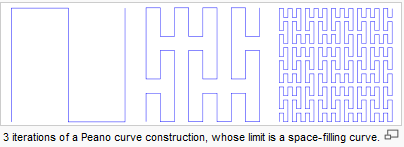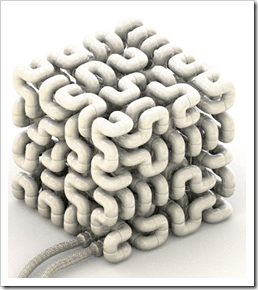## Space-filling curves in theory and practice

In mathematical analysis, a space-filling curve is a curve whose range contains the entire 2-dimensional unit square (or the 3-dimensional unit cube). Because Giuseppe Peano (1858–1932) was the first to discover one, space-filling curves in the 2-dimensional plane are commonly called Peano curves. - WikipediaA practical example of this I found on designboom. See this example of radiator. More details here. Would be a fun project creating a program to do this in AutoCAD.More on space and plane filling curves here and the math behind.Subscribe to the comments feed